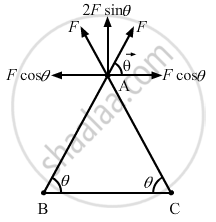Department of Pre-University Education, KarnatakaPUC Karnataka Science Class 12
Advertisement Remove all ads

# Three Equal Charges, 2.0 × 10−6 C Each, Are Held at the Three Corners of an Equilateral Triangle of Side 5 Cm. Find the Coulomb Force Experienced by One of the Charges Due to the Other Two. - Physics

Short Note

Three equal charges, 2.0 × 106 C each, are held at the three corners of an equilateral triangle of side 5 cm. Find the Coulomb force experienced by one of the charges due to the other two.

Advertisement Remove all ads

#### Solution

Since all the charges are of equal magnitude, the force on the charge at A due to the charges at B and C will be of equal magnitude. (As shown in the figure)

That is,

$F_{BA} = F_{CA} = F\left( \text{ say } \right)$The horizontal components of force cancel each other and the net force on the charge at A,

$F' = F_{BA} \sin\theta + F_{CA} \sin\theta$

$F' = 2F\sin\theta$

Given: r = 5 cm =0.05 m
By Coulomb's Law, force,

$F = \frac{1}{4\pi \epsilon_0}\frac{q_1 q_2}{r^2}$

$F' = \frac{2 \times 9 \times {10}^9 \times \left( 2 \times {10}^{- 6} \right)^2 \times \sin60^\circ}{\left( 0 . 05 \right)^2}$

$F' = \frac{2 \times 9 \times {10}^9 \times \left( 2 \times {10}^{- 6} \right)^2 \times \frac{\sqrt{3}}{2}}{\left( 0 . 05 \right)^2}$

F' = 24.9 N

Is there an error in this question or solution?
Advertisement Remove all ads

#### APPEARS IN

HC Verma Class 11, Class 12 Concepts of Physics Vol. 2
Chapter 7 Electric Field and Potential
Q 16 | Page 121
Advertisement Remove all ads

#### Video TutorialsVIEW ALL 

Advertisement Remove all ads
Share
Notifications

View all notifications

Forgot password?